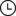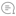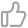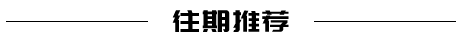超棒！推荐 8 个炫酷的 Python 装饰器！Python学习与数据挖掘 |972021-10-10 09:07000

前言

Python 编程语言的一大优点是它把所有功能都打包到一个小包中，这些功能非常有用。

1. @lru_cache

def factorial(n):
return n * factorial(n-1if n else 1

@lru_cache
def factorial(n):
return n * factorial(n-1if n else 1

2. @jit

JIT 是即时编译(Just In Time)的缩写。通常每当我们在 Python 中运行一些代码时，发生的第一件事就是编译。

Numba JIT 编译器因将这一概念提供到 Python 中而闻名。与@lru_cache 类似，可以非常轻松地调用此装饰器，并立即提高代码的性能。Numba 包提供了 jit 装饰器，它使运行更密集的软件变得更加容易，而不必进入 C。

from numba import jit
import random

@jit(nopython=True)
def monte_carlo_pi(nsamples):
acc = 0
for i in range(nsamples):
x = random.random()
y = random.random()
if (x ** 2 + y ** 2) < 1.0:
acc += 1
return 4.0 * acc / nsamples

3. @do_twice

do_twice 装饰器的功能与它的名字差不多。此装饰器可用于通过一次调用运行两次函数。这当然有一些用途，我发现它对调试特别有用。

from decorators import do_twice
@do_twice
def timerfunc():
%timeit factorial(15)

4. @count_calls

count_calls 装饰器可用于提供有关函数在软件中使用多少次的信息。

from decorators import count_calls
@count_calls
def function_example():
print("Hello World!")

function_example()
function_example()
function_example()

5. @dataclass

from dataclasses import dataclass

@dataclass
class Food:
name: str
unit_price: float
stock: int = 0

def stock_value(self) -> float:
return(self.stock * self.unit_price)

6. @singleton

def singleton(cls):
instances = {}
def wrapper(*args, \*\*kwargs):
if cls not in instances:
instances[cls] = cls(*args, \*\*kwargs)
return instances[cls]
return wrapper

@singleton
class cls:
def func(self):

7. @use_unit

def use_unit(unit):
"""Have a function return a Quantity with given unit"""
use_unit.ureg = pint.UnitRegistry()
def decorator_use_unit(func):
@functools.wraps(func)
def wrapper_use_unit(*args, \*\*kwargs):
value = func(*args, \*_kwargs)
return value _ use_unit.ureg(unit)
return wrapper_use_unit
return decorator_use_unit

@use_unit("meters per second")
def average_speed(distance, duration):
return distance / duration

8. @singledispatch

Functools 凭借非常有用的@singledispatch 装饰器再次在此列表中脱颖而出。

@singledispatch
def fun(arg, verbose=False):
if verbose:
print("Let me just say,", end=" ")
print(arg)

@fun.register
def \_(arg: int, verbose=False):
if verbose:
print("Strength in numbers, eh?", end=" ")
print(arg)

@fun.register
def \_(arg: list, verbose=False):
if verbose:
print("Enumerate this:")
for i, elem in enumerate(arg):
print(i, elem)00

暂无评论~~
Ctrl+Enter